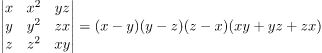# NCERT Solutions Class 12 maths Chapter-4 (Determinants)Exercise 4.2

NCERT Solutions Class 12 Maths from class 12th Students will get the answers of Chapter-4 (Determinants)Exercise 4.2 This chapter will help you to learn the basics and you should expect at least one question in your exam from this chapter.
We have given the answers of all the questions of  NCERT Board Mathematics Textbook in very easy language, which will be very easy for the students to understand and remember so that you can pass with good marks in your examination.

### Set 1

Using the properties of determinants and without expanding in Exercise 1 to 7, prove that:

Question 1Solution:

L.H.S.=C1→C1+C2

=According to Properties of Determinant

=0                                [∵  C& C3 are identical]

Now, L.H.S.=R.H.S.

Hence Proved

Question 2Solution:

L.H.S.==0                        [∵ Every element of C1 are 0]

Now,  L.H.S.=R.H.S.

Hence Proved

Question 3Solution:

L.H.S.=C3→C3-C

===9 ×0=0          [∵C2 & C are identical]

Now,  L.H.S.=R.H.S.

Hence Proved

Question 4Solution:

L.H.S.==Now,  L.H.S.=R.H.S.

Hence Proved

Question 5Solution:

L.H.S.=Now, L.H.S.=R.H.S.

Hence Proved

Question 6Solution:

Let Δ=Taking (-1) common from every row

Δ=(-1)3Interchange rows and columns

Δ=-Now, Δ=-Δ

Δ+Δ=0

2Δ=0

Δ=0

Now,  L.H.S.=R.H.S.

Hence Proved

Question 7Solution:

L.H.S.=Taking common a from Row 1,

b from Row 2,

c from Row 3, we haveNow,  L.H.S.=R.H.S.

Hence Proved

By using properties of determinants, in Exercises 8 to 14, show that:

Question 8(i).(ii)Solution:

(i) L.H.S.=Now,  L.H.S.=R.H.S.

Hence Proved

(ii)  L.H.S.=Now,  L.H.S.=R.H.S.

Hence Proved

Question 9.Solution:

L.H.S.=Now, L.H.S.=R.H.S.

Hence Proved

Question 10.

(i)(ii)Solution:

(i) L.H.S.=Now,  L.H.S.=R.H.S.

Hence Proved

(ii) L.H.S.=Now,  L.H.S.=R.H.S.

Hence Proved

### Set 2

Question 11.

(i)(ii)Solution:

(i) L.H.S.=∵ L.H.S.=R.H.S

Hence proved

(ii)∵ L.H.S.=R.H.S

Hence proved

Question 12Solution:∵ L.H.S.=R.H.S

Hence proved

Question 13Solution:∵ L.H.S.=R.H.S

Hence proved

Question 14Solution:Multiplying column1,column2,column3  by  a,b,c respectively and then dividing the determinant by  a,b,c .∵ L.H.S.=R.H.S

Hence proved

Choose the correct answer in Exercises 15 and 16.

Question 15. Let A be a square matrix of order 3 x 3, then|A| is equal to:

(A) k|A|

(B) k2|A|

(C) k3|A|

(D) 3k|A|

Solution:

Let A =be a square matrix of order 3 x 3   ….(1)

Now, kA=⇒|kA|=⇒|kA|=k3∴ |kA|= k3|A|            [ from eq.(1) ]

∴ option (C) is correct.

Question 16.Which of the following is correct

(A) Determinant is a square matrix.

(B) Determinant is a number associated to a matrix.

(C) Determinant is a number associated to a square matrix.

(D) None of these

Solution:

Since, Determinant is a number which is always associated to a square matrix.

option (C) is correct.